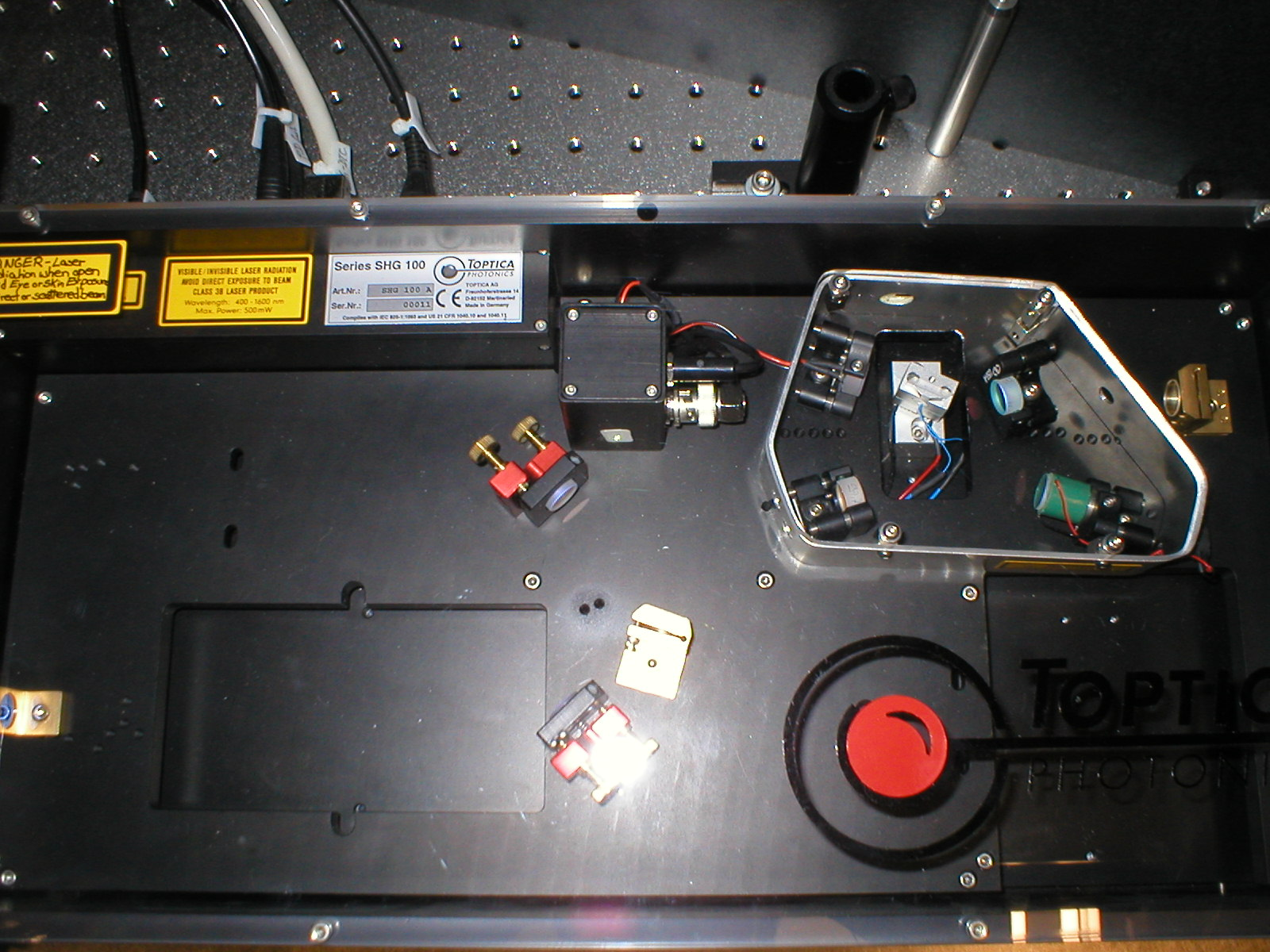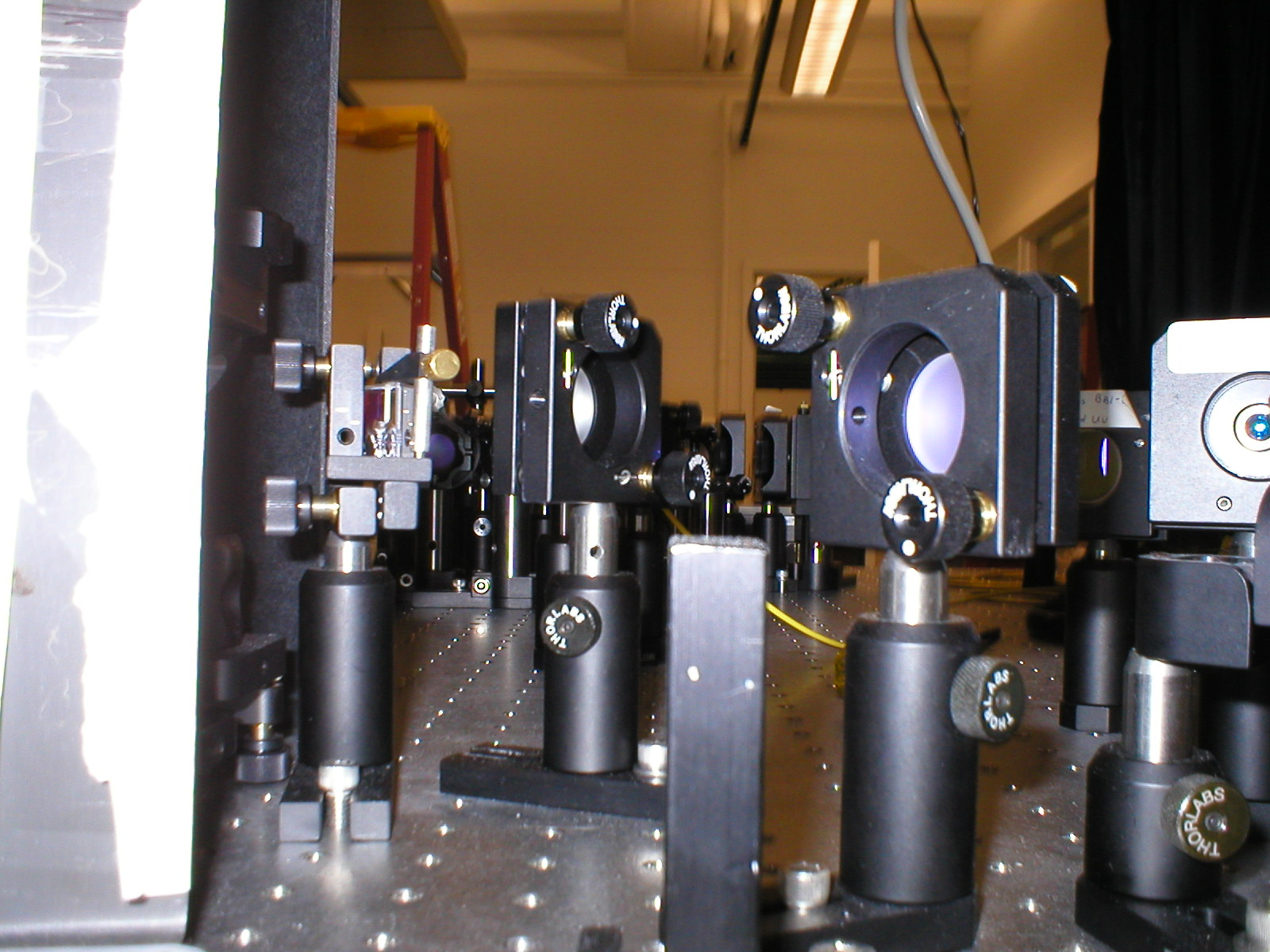Dr. John C. Howell Department of Physics and Astronomy   University of RochesterQuantum Photon Teleportation:

In 1935 Einstein, Podolsky and Rosen theorized a gedonken experiment involving two particles entangled simultaneously over a continuum of position and momentum states.  They decided that if you measure the momentum and position of one particle, based on conservation of momentum you can infer the position and momentum of the other with great certainty.  If one assumes that the particles do not directly interact, then you violate Heisenberg's Uncertainty Principle because you can simultaneously find the position and momentum of the other particle.  This was their paradox and they came to the conclusion that since the particles do not interact, quantum physics in incomplete.  By the mid-1960's J.S.Bell did work leading to his famous inequalities, and proved that quantum physics is without a doubt complete.  Therefore, Einstein's assumption was incorrect, and the paradox was resolved: the entangled particles interact nonlocally.

Our Work:

The idealized entangled state proposed by EPR is one where the position and momentum of two particles are perfectly correlated, and can't be executed in the lab. However, the state of light produced using Parametric Down Conversion can be made to approximated the EPR state.  Parametric Down Conversion is a process in which one "pump" photon is converted into two photons (signal and idler photons) with approximately 1/2 the energy of the original photon.  Also, because of conservation of momentum, Psignal + Pidler = Ppump.  Therefore, if you measure the position or momentum of one, you can infer those of the other.  With parametric down conversion, a demonstration of the EPR paradox has been done using position and momentum entangled photons.  Furthermore, we have found that the position and momentum correlations are strong enough to infer the position or momentum of a photon from that of its partner with a product of variances of ≤ 0.01h2.  (FIND HBAR SYMBOL) This violates the known separability bound by two orders of magnitude.Parametric down conversion is generated by pumping a 2 mm thick type II beta-barium-borate crystal with a 30 mW, 390 nm laser.  The signal and idler photons generated have orthoganal polarizations are then separated by a ploarizing beam splitter.  The apparatus branches into two arms, and in each arm the light passes through a narrow 40 μm vertical slit, a 10 nm spectral filter and a microscope objective that focuses the light onto a multimode fiber coupled to a single photon-counting module. In order to measure correlations in the positions of the entangled photons, a lens of focal length 100 mm is used to image the exit face of the crystal onto planes of the two slits.  One slit is fixed at the location where the signal peaks, and the other is mounted on a translation stage.  the photon coincidence rate is recorded as a function of displacement of the second slit.  In order to measure correlations in the transverse momenta of the photons, the imaging lens is replaced by two lenses of focal length 100 mm, one in each arm, at a distance of the focal length from the planes of the two slits.  This means position correlations are obtained by imaging the birthplace of each pair onto a separated detector, and the momentum correlations are obtained by imaging the propogation direction of each photon pair onto a separate detector.

Based on theory, the variance product should be 0.0036h2.  This is much smaller than the experimentally expected value even though the data appear to match the expected curves.  Because of this 2-order-of-magnitude violation, these results constitute a strong violation of EPR's criterion.  In conclusion, we have realized the EPR paradox using momentum-position entangled photons in a method that is easier and more attractive than the similar squeezed-light methods.

University of Rochester

Physics and Astronomy